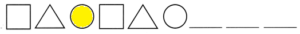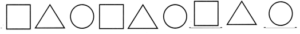# Math in Focus Grade 1 Cumulative Review Chapters 5 and 6 Answer Key

This handy Math in Focus Grade 1 Workbook Answer Key Cumulative Review Chapters 5 and 6 detailed solutions for the textbook questions.

## Math in Focus Grade 1 Cumulative Review Chapters 5 and 6 Answer Key

Concepts and Skills

Look at the picture.

Count and write the number of shapes you see.

Question 1.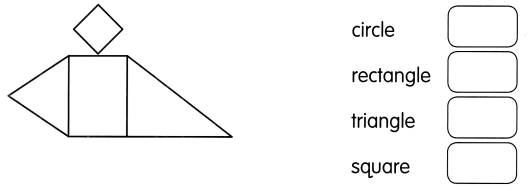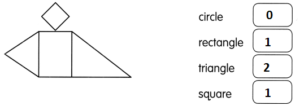Question 2.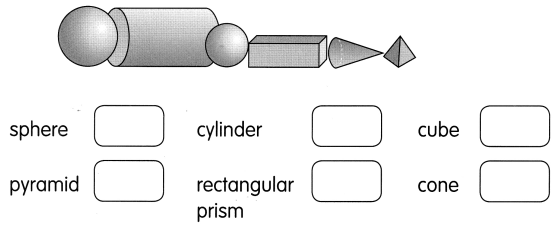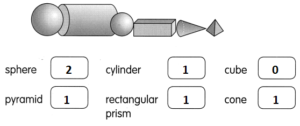Find how many sides and corners.

Question 3.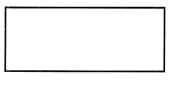__ sides
___ corners
The number of sides are 4.
The number of corners are 4.

Sort the shapes by size.
Color the shapes that are different.

Question 4.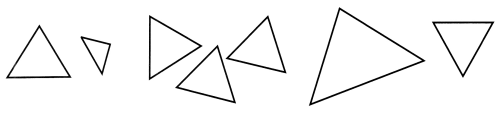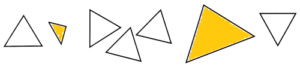Sort the shapes by the number of sides.
Color the shapes that are alike.

Question 5.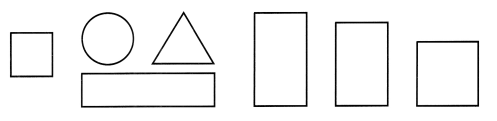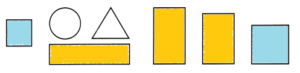Circle the shapes that roll.

Question 6.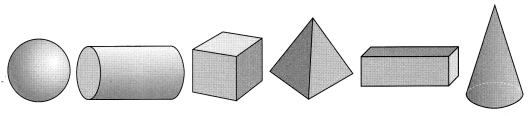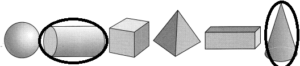Circle the shapes that stack and slide.

Question 7.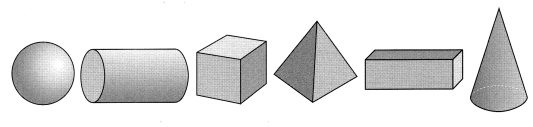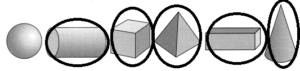Look at the picture.
Circle the correct shape.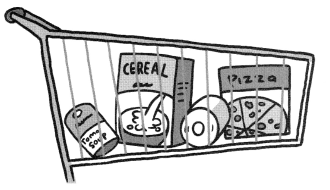Question 8.
The soup can is the shape of a cone/cylinder.
The soup can is the shape of a cylinder.

Question 9.
The cereal box is the shape of a rectangular prism/rectangle.
The cereal box is the shape of a rectangular prism.

Question 10.
The roll of paper towel is the shape of a sphere/cylinder.
The roll of the paper tower is the shape of a cylinder.

Question 11.
The pizza box top is the shape of a cube/square.
The pizza box top is the shape of a square.

Complete the pattern. Circle the missing shape.

Question 12.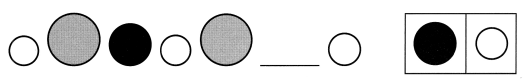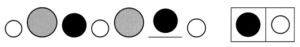Question 13.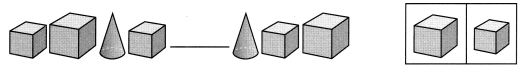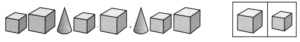Color.

Question 14.
the 3rd sticker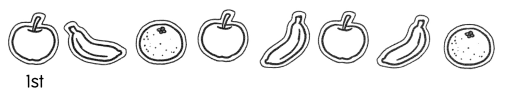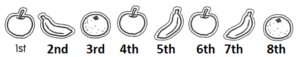Question 15.
the 6th baseball glove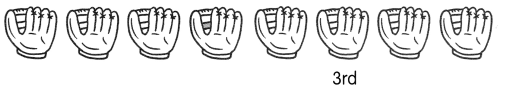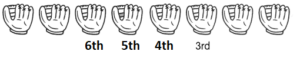Question 16.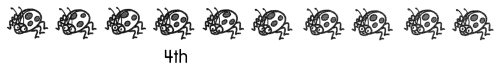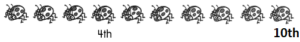Match.

Question 17.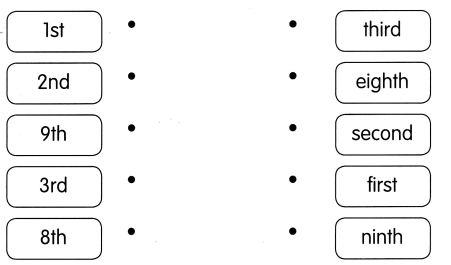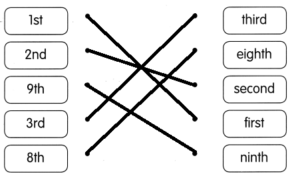Look at each picture. Circle the correct word.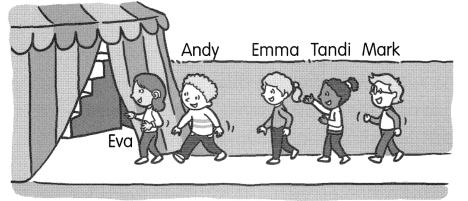Question 18.
Andy is after/before Eva.
Andy is before the Eva.

Question 19.
Emma is before/between Tandi and Mark.
Emma is before the Tandi.

Question 20.
Tandi is after/between Emma and Mark.
Tandi is between Emma and Mark.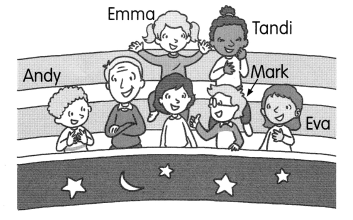Question 21.
Mark is 2nd from the left/right.
Mark is 2nd from the right.

Question 22.
Andy is first/last on the left.
Andy is first on the left.

Question 23.
Mark is in front of/behind Tandi.
Mark is in front of Tandi

Question 24.
Andy is near/far from Eva.
Andy is far from Eva.

Problem Solving

Solve.

Question 25.
Shantel draws a rectangle. Then she draws a line to make two new shapes. The two new shapes are alike. Each new shape is the same shape and size. Each new shape has 3 corners and 3 sides. Draw a line to make the two shapes.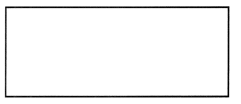Complete.

Question 26.
This is a shape pattern.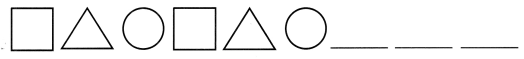a. Color the 3rd shape.